• Introduction
• What is in this manual
• What is Caspoc
• User interface
• Introduction
• Starting
• Simulation
• Editing
• Viewing and printing
• Getting Started
• Basic editing
• Simulation in the time domain
• Basic User Interface Topics
• Editing
• Simulation
• Viewing
• Library
• Reports
• Project management
• Circuit and Block Diagram Components
• Introduction
• Cscript and user defined functions
• Component parameters
• Modeling Topics
• Introduction
• Power Electronics
• Semiconductors
• Electrical Machines
• Electrical drives
• Power Systems
• Mechanical Systems
• Thermal Systems
• Magnetic Circuits
• Green Energy
• Coupling to FEM
• Experimenter
• Analog hardware description language
• Embedded C code Export
• Coupling to Spice
• Small Signal Analysis
• Matlab coupling
• Tips and tricks
• Appendices

## Wind Turbine

Implementation of a model of a variable pitch controlled wind turbine.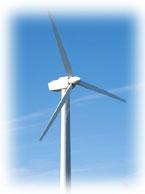The model is based on the steady-state power characteristics of the turbine. The stiffness of the drive train is infinite and the friction factor and the inertia of the turbine must be combined with those of the generator coupled to the turbine. The output power of the turbine is given by the following equation.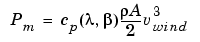where

 Pm Mechanical output power of the turbine (W) cp Performance coefficient of the turbine ρ Air density (kg/m3) A Turbine swept area (m2) vwind Wind speed (m/s) λ Tip speed ratio of the rotor blade tip speed to wind speed β Blade pitch angle (deg)

A generic equation is used to model cp(λ,β). This equation, based on the modeling turbine characteristics of , is: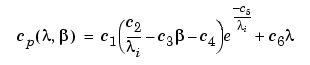with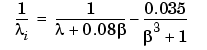The coefficients c1 to c6 are: c1 = 0.5176, c2 = 116, c3 = 0.4, c4 = 5, c5 = 21, c6 = 0.0068, c7 =0.08 and c8 =0.035. The cp-λ characteristics, for different values of the pitch angle β, are illustrated below. The maximum value of cp (cpmax = 0.48) is achieved for β = 0 degree and for λ = 8.1. This particular value of λ is defined as the nominal value (λ_nom).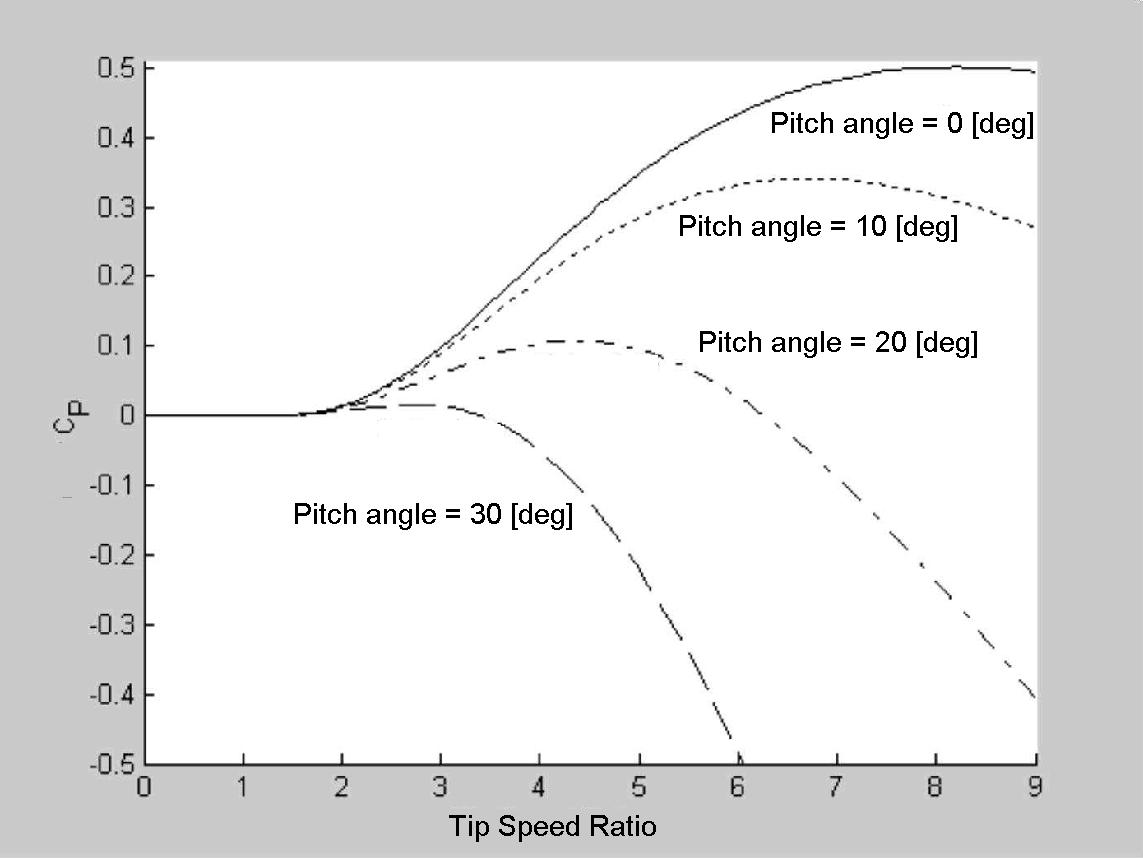Nominal values that are used for calculating λ=vgenerator/vwind are

 vwind_nom Nominal wind speed (m/s) = 12 (m/s) vgenerator_nom Nominal generator speed (rad/s) = ωgenerator*2*π (rad/s) λ_nom Nominal tip speed ratio of the rotor blade tip speed to wind speed = 8.1

Tip speed ration λ=λ_nom * (ωgeneratorgenerator_nom)/(vwind/vwind_nom)

Wind turbine application

In this application a wind turbine is coupled to a synchronous generator. The wind speed is set to a constant value of 12[m/s] The shaft of the wind turbine is coupled to the synchronous generator and the inertia of the shaft is modeled by the extra inertia.
The second input to the wind turbine model is the pitch of the rotor blades. In this application the pitch is set to 0 degrees. It can be connected to a pitch controller, in order to simulate the pitch controller to optimize the power produced by the wind turbine.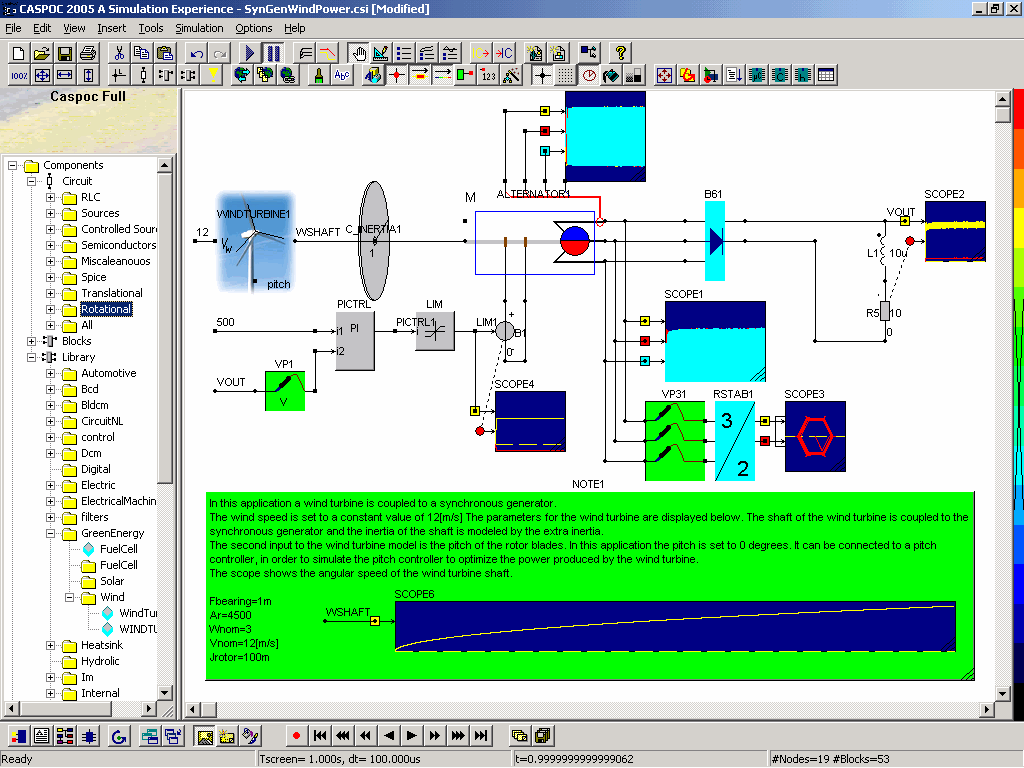Siegfried Heier, Grid Integration of Wind Energy Conversion Systems, John Wiley & Sons Ltd, 1998, ISBN 0-471-97143-X